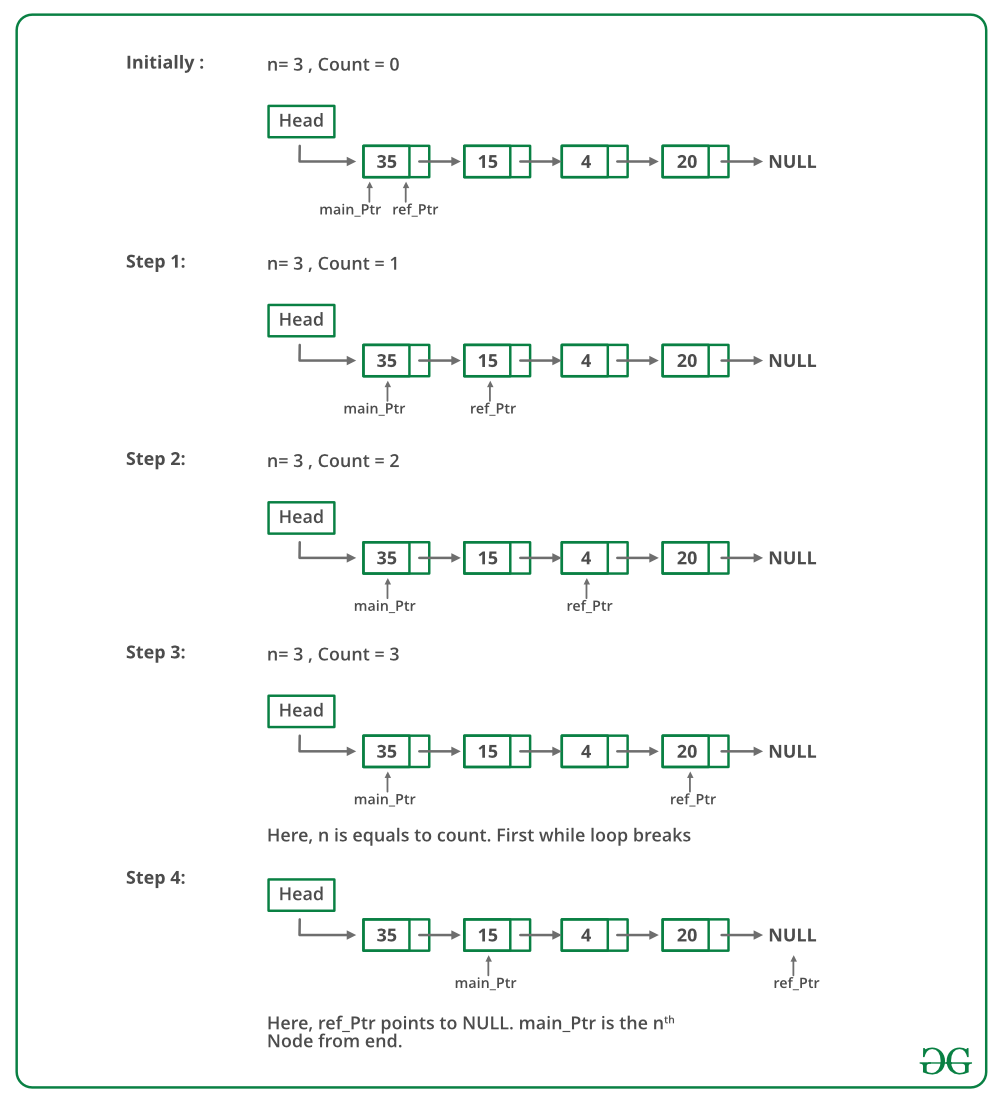GeeksforGeeks App
Open AppBrowser
Continue

# Java Program For Printing Nth Node From The End Of A Linked List(Duplicate)

Given a Linked List and a number n, write a function that returns the value at the n’th node from the end of the Linked List.
For example, if the input is below list and n = 3, then output is “B”Method 1 (Use length of linked list)
1) Calculate the length of Linked List. Let the length be len.
2) Print the (len – n + 1)th node from the beginning of the Linked List.
Double pointer concept : First pointer is used to store the address of the variable and second pointer used to store the address of the first pointer. If we wish to change the value of a variable by a function, we pass pointer to it. And if we wish to change value of a pointer (i. e., it should start pointing to something else), we pass pointer to a pointer.

Below is the implementation of the above approach:

Output

`35`

Following is a recursive C code for the same method. Thanks to Anuj Bansal for providing following code.

## Java

 `static` `void` `printNthFromLast(Node head, ``int` `n)``{``    ``static` `int` `i = ``0``;` `    ``if` `(head == ``null``)``        ``return``;``    ``printNthFromLast(head.next, n);` `    ``if` `(++i == n)``        ``System.out.print(head.data);``}` `// This code is contributed by rutvik_56.`

Time Complexity: O(n) where n is the length of linked list.

Space Complexity: O(n) for call stack

Method 2 (Use two pointers)
Maintain two pointers – reference pointer and main pointer. Initialize both reference and main pointers to head. First, move the reference pointer to n nodes from head. Now move both pointers one by one until the reference pointer reaches the end. Now the main pointer will point to nth node from the end. Return the main pointer.
Below image is a dry run of the above approach:Below is the implementation of the above approach:

Output

`Node no. 4 from last is 35 `

Time Complexity: O(n) where n is the length of linked list.

Space complexity: O(1) since using constant space

Please refer complete article on Program for n’th node from the end of a Linked List for more details!

My Personal Notes arrow_drop_up